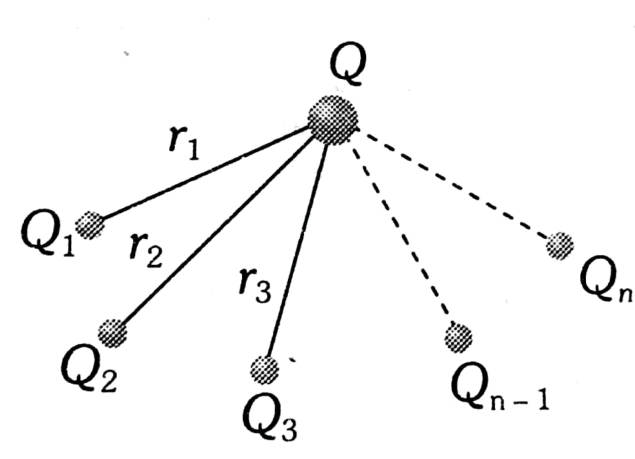# ABC  is a right angled triangle in which AB = 3 cm  and BC = 4 cm . And The three charges +15 , +12   and -20 e.s.u.  are placed respectively on A , B  and C . The force acting on B  is Option 1) Option 2) Option 3) Option 4)

V Vakul

As we learned

Principle of Super Position -

It states that total force acting on a given charge due to number of charges is the Vector sum of the individual force acting on that charge due to all the charges.

- whereinNet force onOption 1)

Option 2)

Option 3)

Option 4)

Exams
Articles
Questions